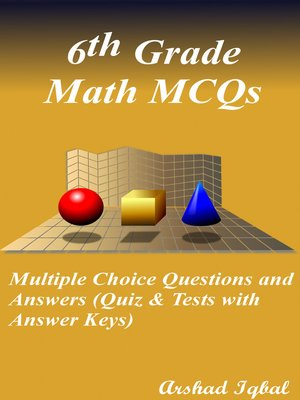## ebook ∣ Quizzes & Practice Tests with Answer Key (Math Quick Study Guides & Terminology Notes about Everything) · Math Quick Study Guides & Terminology Notes about EverythingWith an OverDrive account, you can save your favorite libraries for at-a-glance information about availability. Find out more about OverDrive accounts.

### Title found at these libraries:

6th Grade Math Multiple Choice Questions and Answers (MCQs) PDF: Quiz & Practice Tests with Answer Key (Grade 6 Math Quick Study Guide & Terminology Notes about Everything) includes revision guide for problem solving with 500 solved MCQs. 6th Grade Math MCQ with answers PDF book covers basic concepts, theory and analytical assessment tests. "6th Grade Math MCQ" book PDF helps to practice test questions from exam prep notes.

Math quick study guide provides 500 verbal, quantitative, and analytical reasoning past question papers, solved MCQs. 6th Grade Math Multiple Choice Questions and Answers (MCQs) PDF download, a book to practice quiz questions and answers on chapters: Algebraic equations and simple inequalities, angle properties of polygons, arithmetical problems and percentages, estimation and approximation, factors and multiples, functions and graphs, fundamental algebra, geometrical concepts and properties, integers, number sequences, perimeter and area of geometrical figures, ratio rate and speed, rational numbers, surface area and volume tests for middle school revision guide. 6th Grade Math Quiz Questions and Answers PDF download with free sample test covers beginner's questions, textbook's study notes to practice tests.

Math practice tests with answer key includes primary school worksheets for self-assessment competitive exams. 6th Grade Math MCQ book, a quick study guide with chapter-wise tests for certification exam prep. "6th Grade Math MCQs" PDF book covers problem solving tests from math textbook's chapters as:

Chapter 1: Algebraic Equations and Simple Inequalities MCQs
Chapter 2: Angle Properties of Polygons MCQs
Chapter 3: Arithmetical Problems and Percentages MCQs
Chapter 4: Estimation and Approximation MCQs
Chapter 5: Factors and Multiples MCQs
Chapter 6: Functions and Graphs MCQs
Chapter 7: Fundamental Algebra MCQs
Chapter 8: Geometrical Concepts and Properties MCQs
Chapter 9: Integers MCQs
Chapter 10: Number Sequences MCQs
Chapter 11: Perimeter and Area of Geometrical Figures MCQs
Chapter 12: Ratio Rate and Speed MCQs
Chapter 13: Rational Numbers MCQs
Chapter 14: Surface Area and Volume MCQs

Solve "Algebraic Equations and Simple Inequalities MCQ" book PDF, chapter 1 to solve MCQ questions and answers: Equations and inequalities, examples of equations, inequalities learning, making formula, math formulas, problem solving with algebra, simple equations solutions, solving simple equations, and writing algebraic expressions.

Solve "Angle Properties of Polygons MCQ" book PDF, chapter 2 to solve MCQ questions and answers: Convex polygons, polygons, and types of triangles.

Solve "Factors and Multiples MCQ" book PDF, chapter 5 to solve MCQ questions and answers: Cubes and cube roots, factors and multiples, highest common factor, index notation, least common multiple, prime and composite numbers, prime factorization, squares and square roots.

Solve "Functions and Graphs MCQ" book PDF, chapter 6 to solve MCQ questions and answers: Cartesian plane, finding coordinates, and idea of functions.

Solve "Fundamental Algebra MCQ" book PDF, chapter 7 to solve MCQ questions and answers: Algebra rules, algebraic expressions: addition and subtraction, algebraic notation, brackets, factorization, evaluation of algebraic expressions, factorization by grouping, linear algebraic expressions and fractional coefficients, and writing algebraic expressions.

Solve "Geometrical Concepts and Properties MCQ" book PDF, chapter 8 to solve MCQ questions and answers: Adjacent angles, Cartesian plane, complementary angles, geometric concepts, line rays...